# C++ Basics | Data Types | Variables

## C++ Basics | Data Types | Variables

In this article, I am going to discuss the basics of the C++ Program. Please read our previous article where we discussed how to set up the C++ environment, how to download compiler, IDE, etc. In this article, we are going to write our first C++ program also we are going to discuss what are the basic syntax that is needed to write the first program. Topics covered under this article.

1. Skeleton of C++ program
2. Welcome to the C++ program
3. What and why data types?
4. Primitive data types
5. Variables
##### Skeleton of C++ program

To write a C++ program and to execute successfully we need to follow some standard procedure that is specific to C++ and each language will have their own procedure/rules. The basic C++ program skeleton looks like this.

```#include<iostream>
int main()
{
//program or logic;
return 0;
}
```

This is the most basic thing, which is needed to write and execute a C++ program. There are many unanswered questions in your mind if you are new to programming. What exactly #include <iostream> means why we need it etc…

##### What is #include?

#include is a preprocessor directive that asks the compiler to include the header file mentioned within the ‘< >’ or within the “ “ quotes. Here in the above C++ skeleton, we are requesting the compiler to include iostream header file. (Iostream stands for input/output stream, which is responsible for basic input/output operation). These iostream header files are also programs, which are already written and known to C++ compilers.

##### What is int main()?

Basically, int main is a method as the name itself suggests it is the main method or the first method which gets called when you run the C++ program. Actual program execution starts from main() method.

##### Why return 0?

It is an information to Operating System program terminated successfully. It is like a standard in C++ programs, it must be written. return 0;

##### First C++ Program

Let us write the first program in C++.

```#include<iostream>
using namespace std;
int main()
{
cout<<"welcome to C++"<<endl;
return 0;
}
```

If you are not using the IDE to compile this program you need to execute the following command.
g++ -o exe_filename yourfilename.cpp
Example: g++ -o welcome welcome.cpp

To run the program, execute the following command.
./exe_filename
Example: ./welcome

Note: If you are not giving executable file name then default file name while Compiling explicitly then the compiler will choose default filename a.out.

If you are using IDE check for the build and run icon and click on it. I am showing devc++ for all my explanations will show the shortcuts also.

1. To Build or compile the program: F9
2. To Run the Program: F10
3. To build and run the program: F11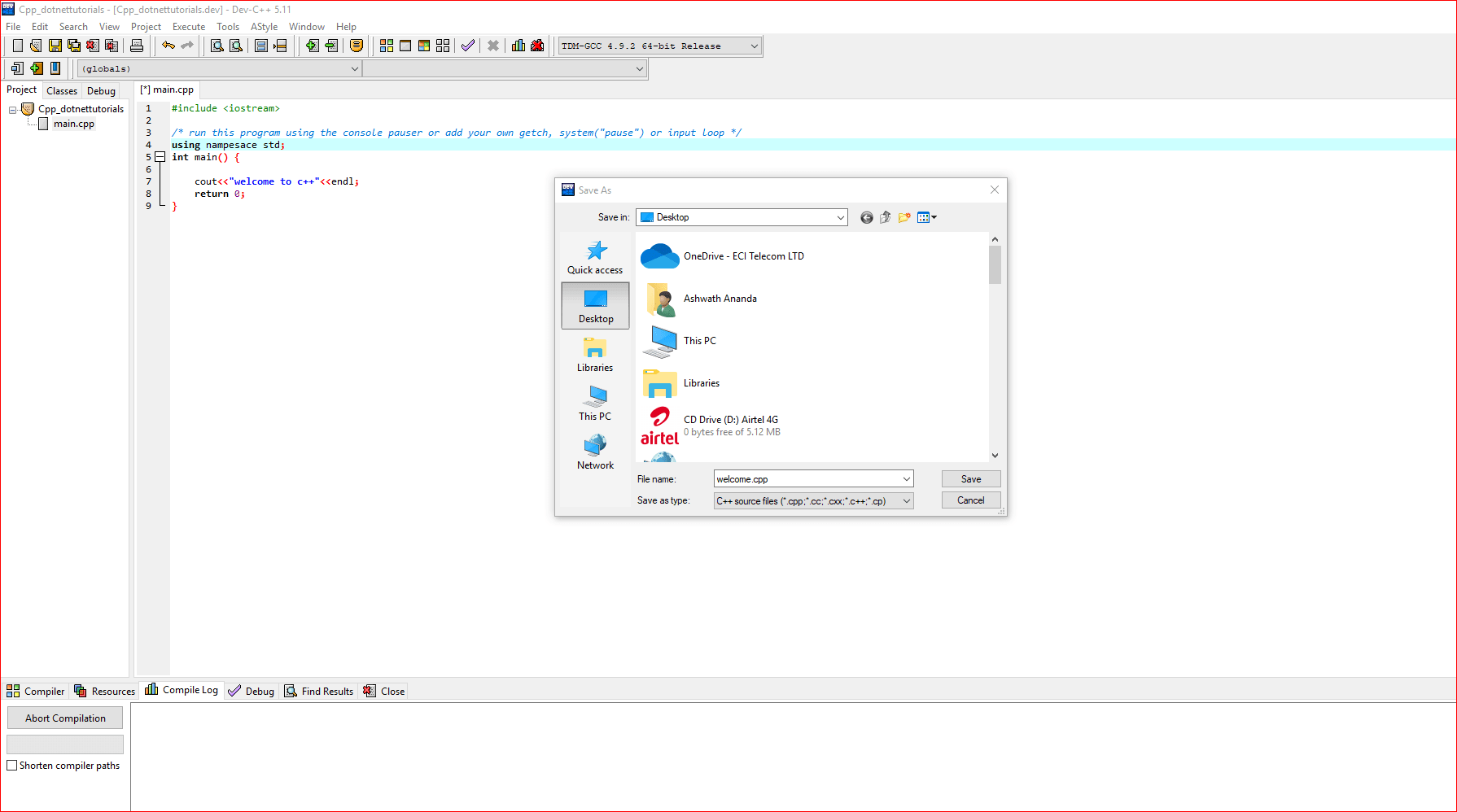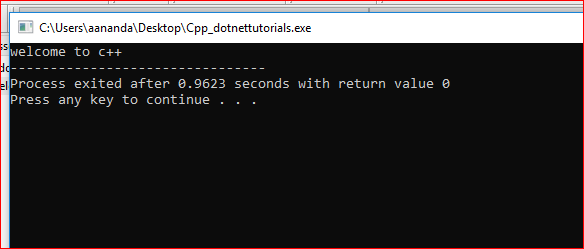In IDE internally g++ compiler will be called to compile the program.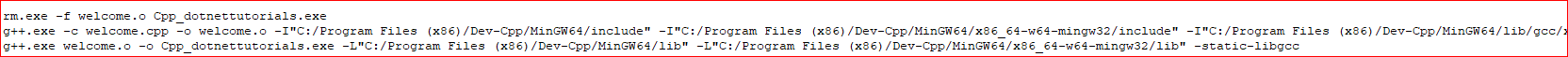I already explained why we need #include, main, and return. Now I will be discussing what is this using namespace std, what is cout?

##### What is cout in C++?

Cout is nothing but console output using this we can display the content in the terminal. Similar to cout there are standard streams each will be used to serve a different purpose.

 cin standard input stream cerr standard error (output) stream clog standard logging (output) stream
##### What is using namespace std?

C++ supports a concept called namespace, which will be discussed in detail in coming lectures where all the built-in functions and objects are present. std namespace contains cout, cin, and other functions. To use this in our program we are informing the compiler by saying using namespace std. There is another way of writing it.

std::cout<<“welcome to c++”<<endl;// if we have written like this then it not required to mention using namespace std at the beginning of the program.

##### What are Data Types and Why we need Data Types in C++?

Program or instruction always acts on Data. Let’s explain this in detail. Usually, the main memory is divided into the code section, stack, heap section.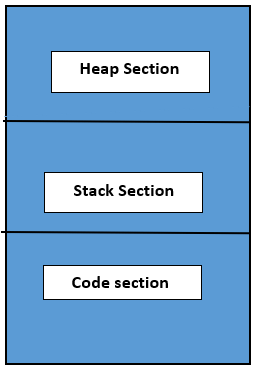Whenever we execute the program all the instruction is loaded into the code section and data is loaded into the stack section/heap section depends on where exactly we need to store the data but usually, data is stored in the stack section.

Now let’s talk about data types. As I said earlier program acts on data but program logic depends on what type of data we are manipulating or handling.

Usually, Data can be of two types. Numeric data and character or alphabets data. If you are interested in performing arithmetic operation then we usually using and manipulating numeric data.

Example of numeric data:10,10.5,01010,0X10 etc.

If you are interested in storing, sorting employee names, etc. then we usually using character or string data.

Example: ‘A’, “Ashwath”, “Ash26”, etc.

##### Now let us answer why we need Data Types.

We need Data types to inform the Operating System program what is the type of data we are handling based on the type of data it will allocate memory in Bytes in the main memory for the particular Data Types. Let us discuss the data types available in C++.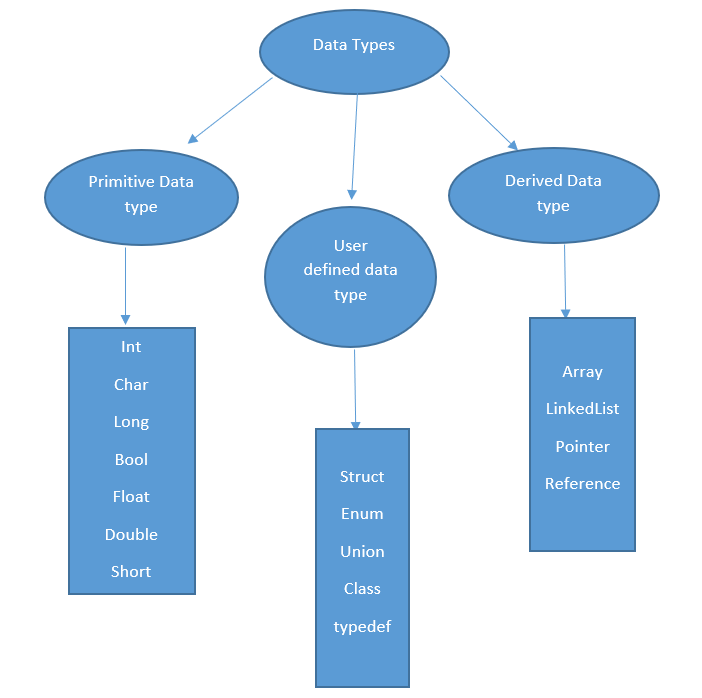High-level classification of Data types each will be discussed in detail in future articles.

##### Primitive Data Types:

These are the Basic data types provided or available in C++. We can call it as built-in data types. Let’s categorize further.

1. Integer data type: int, short, long
2. Floating point data type: Float, double
3. Boolean data type: bool
4. character data type: char

Each data type occupies a different size in memory. Instead of remembering how much byte int, char, float occupies let’s write a program to identify the size of each data type.

```#include <iostream>
using namespace std;
int main ()
{
cout << "size of int data type:\t" << sizeof (int) << endl;
cout << "size of long data type:\t" << sizeof (long) << endl;
cout << "size of char data type:\t" << sizeof (char) << endl;
cout << "size of bool data type:\t" << sizeof (bool) << endl;
cout << "size of float data type:\t" << sizeof (float) << endl;
cout << "size of double data type:\t" << sizeof (double) << endl;
cout << "size of short data type:\t" << sizeof (short) << endl;
return 0;
}
```

Output: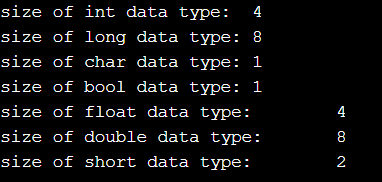Note: using sizeof() method we can identify the number of bytes each data type occupies in the main memory.

##### Variables:

Variables are the name given to the memory location where we store the data. Variable as the same meaning as that of maths. x-5=0 here x is a variable whose value is 5 means x is nothing but 5 or x is equal to 5. Similarly, in the C++ variable is used to store the values it may be an integer value, character value, or anything.

Ax+bx+c=0 in math, here only x is considered as variable, and other is considered as constants similar to qualify a variable is a valid one or not, to declare a variable there is a rule. I will tell what are the valid variables which we can use in our program and what we can’t use as variables in our program.

##### Valid variables:
1. Variable names should be from 1 to 255 characters in length.
2. A variable name should be beginning with the alphabets or underscore ‘_’.

Example: ash, _ash, a23, A41, A2ds

##### Invalid variables:
1. We cannot use the keyword as a variable name.
2. We cannot use spaces or a special character as a variable name.

Example: int, const, ash wath, ash@sdf! Etc. are examples of invalid variable names.

In the next article, we are going to discuss Arithmetic operators, Associativity, logical operators, compound assignment. Here, in this article, I try to explain the basics of the C++ Program and I hope you enjoy this article.